Search

# Python Data Science Homework Help | Data Preparation Part - 1 | Realcode4you

In this blog we will learn all the basic topics of python data science.

### Data preparation

1. Introducing pandas

2. Series

3. DataFrames

4. Missing Data

5. GroupBy

6. Merging, Joining and Concatenating

7. Operations

8. Data Input and Output

### 1. Introducing pandas

Pandas is a Python library that makes handling tabular data easier. Since we're doing data science - this is something we'll use from time to time!

It's one of three libraries you'll encounter repeatedly in the field of data science:

Pandas

Introduces "Data Frames" and "Series" that allow you to slice and dice rows and columns of information.

NumPy

Usually you'll encounter "NumPy arrays", which are multi-dimensional array objects. It is easy to create a Pandas DataFrame from a NumPy array, and Pandas DataFrames can be cast as NumPy arrays. NumPy arrays are mainly important because of...

Scikit_Learn

The machine learning library we'll use throughout this course is scikit_learn, or sklearn, and it generally takes NumPy arrays as its input.

So, a typical thing to do is to load, clean, and manipulate your input data using Pandas. Then convert your Pandas DataFrame into a NumPy array as it's being passed into some Scikit_Learn function. That conversion can often happen automatically.

Let's start by loading some comma-separated value data using Pandas into a DataFrame: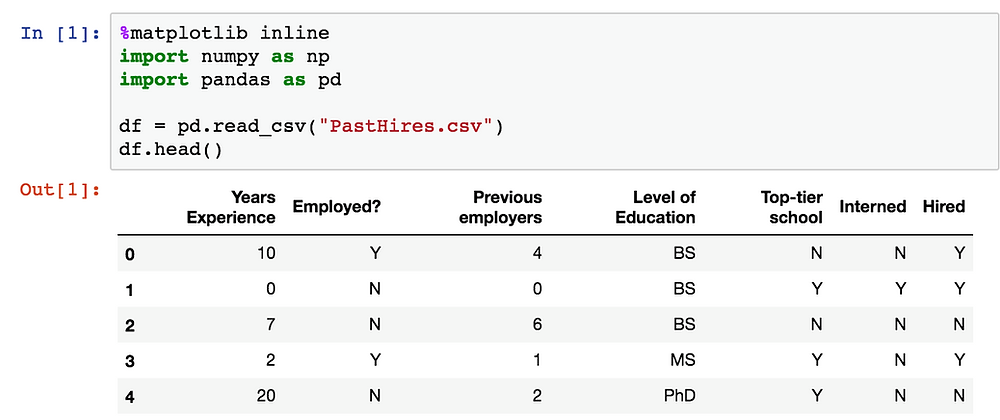head() is a handy way to visualize what you've loaded. You can pass it an integer to see some specific number of rows at the beginning of your DataFrame:You can also view the end of your data with tail():We often talk about the "shape" of your DataFrame. This is just its dimensions. This particular CSV file has 13 rows with 7 columns per row:The total size of the data frame is the rows * columns:The len() function gives you the number of rows in a DataFrame:If your DataFrame has named columns (in our case, extracted automatically from the first row of a .csv file,) you can get an array of them back:Extracting a single column from your DataFrame looks like this - this gives you back a "Series" in Pandas: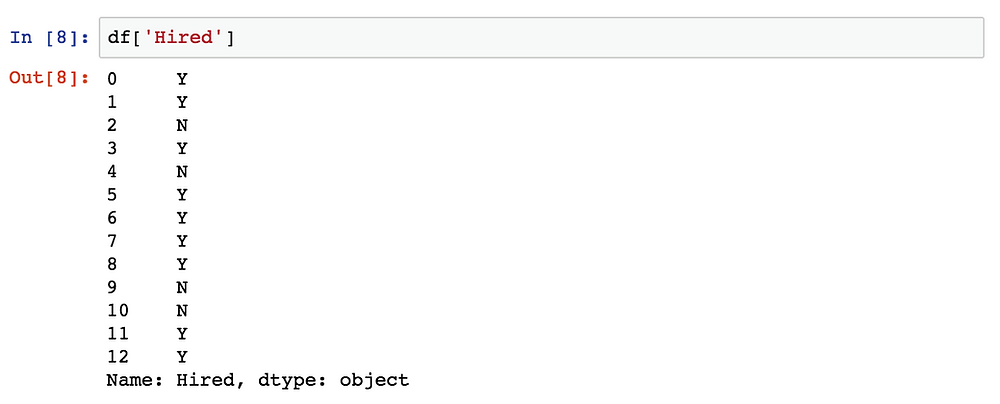You can also extract a given range of rows from a named column, like so:Or even extract a single value from a specified column / row combination:To extract more than one column, you pass in a list of column names instead of a single one:You can also extract specific ranges of rows from more than one column, in the way you'd expect:Sorting your DataFrame by a specific column looks like this:You can break down the number of unique values in a given column into a Series using value_counts() - this is a good way to understand the distribution of your data:Pandas even makes it easy to plot a Series or DataFrame - just call plot():### 2. Series

The first main data type we will learn about for pandas is the Series data type. Let's import Pandas and explore the Series object.

A Series is very similar to a NumPy array (in fact it is built on top of the NumPy array object). What differentiates the NumPy array from a Series, is that a Series can have axis labels, meaning it can be indexed by a label, instead of just a number location. It also doesn't need to hold numeric data, it can hold any arbitrary Python Object.

Let's explore this concept through some examples2.1 Creating a Series

You can convert a list,numpy array, or dictionary to a Series: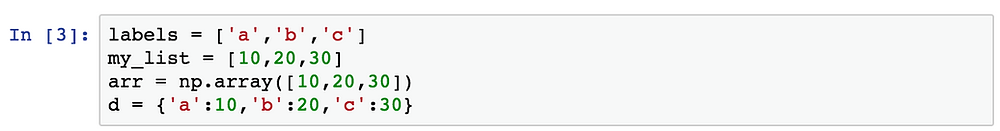Using ListsNumPy ArraysDictionary2.2 Data in Series

A pandas Series can hold a variety of object types: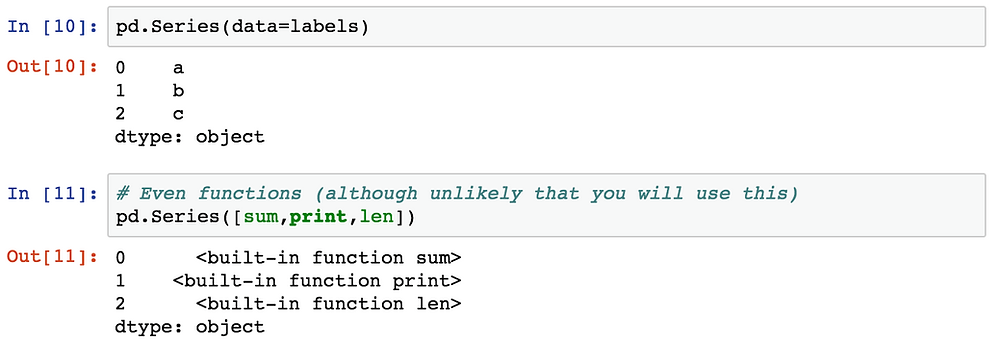2.3 Using an index

The key to using a Series is understanding its index. Pandas makes use of these index names or numbers by allowing for fast look ups of information (works like a hash table or dictionary).

Let's see some examples of how to grab information from a Series. Let us create two sereis, ser1 and ser2:Operations are then also done based off of index:Let's stop here for now and move on to DataFrames, which will expand on the concept of Series!

### 3. DataFrames

DataFrames are the workhorse of pandas and are directly inspired by the R programming language. We can think of a DataFrame as a bunch of Series objects put together to share the same index. Let's use pandas to explore this topic!3.1 Selection and Indexing

Let's learn the various methods to grab data from a DataFrameDataFrame Columns are just SeriesCreating a new column:Removing Columns: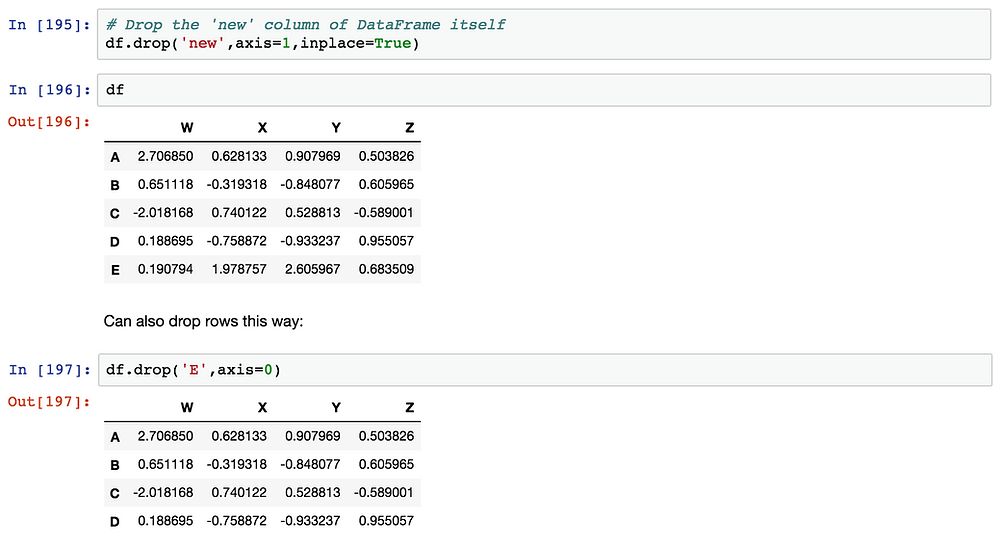### Other Assignment realted help serives which offered by us

<Realcode4you> Assignment Help

<Realcode4you> Web Assignment Help

In next part we will covers all the remaining topics of data science preparation, I hope it may helpful to learning the data science. We will also offers the assignment, project and programming help services in all the programming languages, if you need any assignement related help then contact us directly here